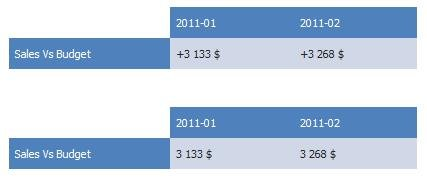In table settings, it’s possible to show positive values with a + as a prefix (negative values are always shown with a – as a prefix in Dapresy Pro). The setting option is useful when a table for example shows a “difference” between two values, where the difference has been calculated in a computed variable or imported via the data file as in those cases the normal system behavior is to not show the result with a + as the system does not recognize the results as a difference between two values.

The example tables below shows a typical use case where the difference between Sales and Budget has been calculated in a Computed variable, it is useful to show a + as a prefix in this case. The top table uses the settings option, while the bottom table does not use this option.